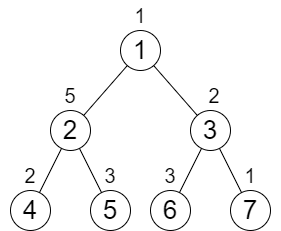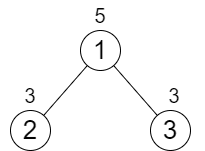# 2673. Make Costs of Paths Equal in a Binary Tree

## Description

You are given an integer n representing the number of nodes in a perfect binary tree consisting of nodes numbered from 1 to n. The root of the tree is node 1 and each node i in the tree has two children where the left child is the node 2 * i and the right child is 2 * i + 1.

Each node in the tree also has a cost represented by a given 0-indexed integer array cost of size n where cost[i] is the cost of node i + 1. You are allowed to increment the cost of any node by 1 any number of times.

Return the minimum number of increments you need to make the cost of paths from the root to each leaf node equal.

Note:

• A perfect binary tree is a tree where each node, except the leaf nodes, has exactly 2 children.
• The cost of a path is the sum of costs of nodes in the path.

Example 1:Input: n = 7, cost = [1,5,2,2,3,3,1]
Output: 6
Explanation: We can do the following increments:
- Increase the cost of node 4 one time.
- Increase the cost of node 3 three times.
- Increase the cost of node 7 two times.
Each path from the root to a leaf will have a total cost of 9.
The total increments we did is 1 + 3 + 2 = 6.
It can be shown that this is the minimum answer we can achieve.


Example 2:Input: n = 3, cost = [5,3,3]
Output: 0
Explanation: The two paths already have equal total costs, so no increments are needed.


Constraints:

• 3 <= n <= 105
• n + 1 is a power of 2
• cost.length == n
• 1 <= cost[i] <= 104

## Solutions

• class Solution {
private int[] cost;
private int n;
private int ans;

public int minIncrements(int n, int[] cost) {
this.n = n;
this.cost = cost;
dfs(1);
return ans;
}

private int dfs(int i) {
if ((i << 1) > n) {
return cost[i - 1];
}
int l = dfs(i << 1);
int r = dfs(i << 1 | 1);
ans += Math.max(l, r) - Math.min(l, r);
return cost[i - 1] + Math.max(l, r);
}
}

• class Solution {
public:
int minIncrements(int n, vector<int>& cost) {
int ans = 0;
function<int(int)> dfs = [&](int i) -> int {
if ((i << 1) > n) {
return cost[i - 1];
}
int l = dfs(i << 1);
int r = dfs(i << 1 | 1);
ans += max(l, r) - min(l, r);
return cost[i - 1] + max(l, r);
};
dfs(1);
return ans;
}
};

• class Solution:
def minIncrements(self, n: int, cost: List[int]) -> int:
def dfs(i: int) -> int:
if (i << 1) > n:
return cost[i - 1]
l, r = dfs(i << 1), dfs(i << 1 | 1)
nonlocal ans
ans += max(l, r) - min(l, r)
return cost[i - 1] + max(l, r)

ans = 0
dfs(1)
return ans


• func minIncrements(n int, cost []int) (ans int) {
var dfs func(int) int
dfs = func(i int) int {
if (i << 1) > n {
return cost[i-1]
}
l, r := dfs(i<<1), dfs(i<<1|1)
ans += max(l, r) - min(l, r)
return cost[i-1] + max(l, r)
}
dfs(1)
return ans
}

func max(a, b int) int {
if a > b {
return a
}
return b
}

func min(a, b int) int {
if a < b {
return a
}
return b
}

• function minIncrements(n: number, cost: number[]): number {
let ans = 0;
const dfs = (i: number): number => {
if (i << 1 > n) {
return cost[i - 1];
}
const [a, b] = [dfs(i << 1), dfs((i << 1) | 1)];
ans += Math.max(a, b) - Math.min(a, b);
return cost[i - 1] + Math.max(a, b);
};
dfs(1);
return ans;
}